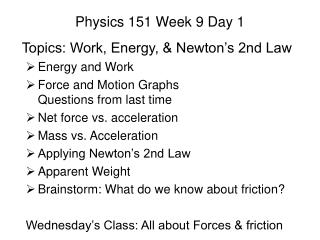# Physics 151 Week 9 Day 1 - PowerPoint PPT PresentationDownload PresentationPhysics 151 Week 9 Day 1

Physics 151 Week 9 Day 1Download Presentation## Physics 151 Week 9 Day 1

- - - - - - - - - - - - - - - - - - - - - - - - - - - E N D - - - - - - - - - - - - - - - - - - - - - - - - - - -
##### Presentation Transcript

1. Physics 151 Week 9 Day 1 Topics: Work, Energy, & Newton’s 2nd Law Energy and Work Force and Motion Graphs Questions from last time Net force vs. acceleration Mass vs. Acceleration Applying Newton’s 2nd Law Apparent Weight Brainstorm: What do we know about friction? Wednesday’s Class: All about Forces & friction

2. Slide 10-3

3. Slide 10-4

4. Forms of Energy Mechanical Energy Thermal Energy Other forms include Slide 10-12

5. The Basic Energy Model Slide 10-13

6. Energy Transformations Kinetic energy K = energy of motion Potential energy U = energy of position Thermal energy Eth = energy associated with temperature System energy E = K + U + Eth + Echem + ... Energy can be transformed within the system without loss. Energy is a property of a system. Slide 10-14

7. Some Energy Transformations Echem Ug KEth Us KUg Echem Ug Slide 10-15

8. Energy Transfers These change the energy of the system. Interactions with the environment. Work is the mechanical transfer of energy to or from a system via pushes and pulls. Slide 10-20

9. Energy Transfers: Work W K W Eth W Us Slide 10-21

10. The Work-Energy Equation Slide 10-22

11. Work Slide 10-28

12. General Force Model • Newton 0th Law • Objects are dumb - They have no memory of the past and cannot predict the future. Objects only know what is acting directly on them right now • Newton's 1st Law • An object that is at rest will remain at rest and an object that is moving will continue to move in a straight line with constant speed, if and only if the sum of the forces acting on that object is zero. • Newton's 3rd Law • Recall that a force is an interaction between two objects. If object A exerts a force on object B then object B exerts a force on object that is in the opposite direction, equal in magnitude, and of the same type. • Visualizations: • Force Diagrams • System Schema

13. Constant Force Model Newton's 2nd Law acceleration of an object = sum of forces acting on that object / the mass of the object Remainder of week: Friction Model Apparent Weight Slide 4-19

14. Net Force and Motion Graphs

15. Net Force vs. Acceleration Graphs

16. Net Force vs. Mass Graphs

17. Reading Quiz • Which of the following statements about mass and weight is correct? • Your mass is a measure of the force gravity exerts on you. • Your mass is the same everywhere in the universe. • Your weight is the same everywhere in the universe. • Your weight is a measure of your resistance of being accelerated. Slide 5-5

18. Answer • Which of the following statements about mass and weight is correct? • Your mass is a measure of the force gravity exerts on you. • Your mass is the same everywhere in the universe. • Your weight is the same everywhere in the universe. • Your weight is a measure of your resistance of being accelerated. Slide 5-6

19. Example Problem • A 100 kg block with a weight of 980 N hangs on a rope. Find the tension in the rope if • the block is stationary. • it’s moving upward at a steady speed of 5 m/s. • it’s accelerating upward at 5 m/s2. Slide 5-15

20. Example Problem A sled with a mass of 20 kg slides along frictionless ice at 4.5 m/s. It then crosses a rough patch of snow which exerts a friction force of 12 N. How far does it slide on the snow before coming to rest? Slide 5-21

21. Example Problem A 75 kg skier starts down a 50-m-high, 10° slope on frictionless skis. What is his speed at the bottom? Slide 5-27

22. Friction Brainstorm One person in each team takes out a sheet of paper and records their group brainstorming everything they know about friction.

23. Example Problem Burglars are trying to haul a 1000 kg safe up a frictionless ramp to their getaway truck. The ramp is tilted at angle θ. What is the tension in the rope if the safe is at rest? If the safe is moving up the ramp at a steady 1 m/s? If the safe is accelerating up the ramp at 1 m/s2? Do these answers have the expected behavior in the limit θ→ 0° and θ→ 90°? Slide 5-28

24. Example Problem Macie pulls a 40 kg rolling trunk by a strap angled at 30° from the horizontal. She pulls with a force of 40 N, and there is a 30 N rolling friction force acting on trunk. What is the trunk’s acceleration? Slide 5-22